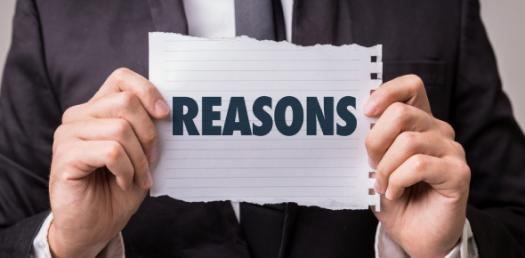# Reasoning (Coding-decoding) Quiz-1

20 Questions | Attempts: 2023
ShareSettings• 1.
In a certain code, LUTE is written as MUTE and GATE is written as HATE, then how will be BLUE be written in that code?
• A.

CLUE

• B.

GLUE

• C.

FLUD

• D.

FLUE

• E.

None of these

• 2.
In a certain code, APPLE is written as BPPLD and CEAT is written as DEAS, then how will be HEART written in that code?
• A.

GEARS

• B.

IEARS

• C.

HEAST

• D.

TREEH

• E.

None of these

• 3.
In a certain code, CAT is written as SATC and DEAR is written as QEARD. How would SING be written in that code?
• A.

BINGS

• B.

SGNIS

• C.

SINGS

• D.

GNISS

• E.

None of these

• 4.
In a certain code language, STRAIN is written as UWTDKQ. How will be PLACES written in that code?
• A.

ROCFGV

• B.

RNDFGV

• C.

RODEGV

• D.

ROCFHV

• E.

None of these

• 5.
In a certain code language, ANTICIPATION is written as ICITNANOITAP. How will be PRODUCTIVITY written in that code?
• A.

CUDORPYTIVTI

• B.

CUDORPYTIUIT

• C.

CODOPRYTIVIT

• D.

CUDORPTYIUIT

• E.

None of these

• 6.
In a certain code language, the word FILAMENT is written as VPGOHKMC. How will be the word CONJUGAL written in that code?
• A.

NCIWLPQE

• B.

NCIWEQPL

• C.

NCWILPQE

• D.

NCIEWPQL

• E.

None of these

• 7.
In a certain code language, GOVERNMENT is written as PIXOTFOUP. How will be the word SHORTAGE written in that code language?
• A.

IUSQBFVI

• B.

IUSQBVFI

• C.

USQAVFI

• D.

JIUSJBFJ

• E.

None of these

• 8.
In a certain code language, FREEDOM is written as GQFDENN. How will be the world STRONGER written in that code language?
• A.

TSSNOFFQ

• B.

TSNSOFQF

• C.

TMSSOFFQ

• D.

TMSSFFOQ

• E.

None of these

• 9.
In a certain code, ZOOM is written as POON and ROAD is written QOBE. How would NOMP be coded in that language?
• A.

PONX

• B.

QOHB

• C.

XONY

• D.

MONZ

• E.

None of these

• 10.
In a certain code, STOVE is written as FNBLK, then how will VOTES be written in the same code?
• A.

FNKBN

• B.

LBNKF

• C.

LKNBF

• D.

LNBKF

• E.

None of these

• 11.
In a certain code language FIRE is written as #%@\$ and DEAL is written as ©\$U. How will FAIL be written in that code language?
• A.

%*U#

• B.

#U%*

• C.

#U%#

• D.

U#*%

• E.

None of these

• 12.
In a certain code language FUEL is written as \$%#6 and KITE is written as @•7#. How will LIFE be written in that code language?
• A.

6•\$#

• B.

6%#\$

• C.

6\$•#

• D.

#6\$•

• E.

None of these

• 13.
In a certain code language MODERN is written as 5%38#@ and WIN is written as 6©@. How will “RIDER” be written inthat code language?
• A.

• B.

• C.

• D.

• E.

None of these

• 14.
In a certain code language TIMES is written as 79548. How will INDIA be written in that code language?
• A.

18132

• B.

45989

• C.

94598

• D.

49589

• E.

None of these

• 15.
In a certain code language, SAFER is written as [email protected]#2 and RIDE is written as 2©%#, how would FEDS be written in that code?
• A.

• B.
• C.

3#%5

• D.

3#%2

• E.

None of these

• 16.
If in a certain code language, EAT is written as 318 and CHAIR is written as 24156, then how TEACHER be written in that code language?
• A.

8313426

• B.

8312436

• C.

8321436

• D.

8312346

• E.

None of these

• 17.
In a certain code RAID is written as %#*\$, RIPE is written as %*@©. How is DEAR written up in that code?
• A.

• B.
• C.

@\$#%

• D.

• E.

None of these

• 18.
In a certain code, DOWN is written as [email protected]# and NAME is written as #6%3. How would MODE be written in that code?
• A.

%653

• B.

%@63

• C.
• D.

%@53

• E.

None of these

• 19.
If GO=32, SHE=49, then SOME will be equal to
• A.

56

• B.

58

• C.

62

• D.

64

• E.

None of these

• 20.
If AT=20, BAT=40, then  CAT will be equal to
• A.

30

• B.

50

• C.

60

• D.

70

• E.

None of these

## Related TopicsBack to top
×

Wait!
Here's an interesting quiz for you.